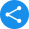# NCERT Book for Class 11 MathsView Notes

## CBSE Class 11 NCERT Books for Maths PDF - Free Download

The National Council of Educational Research and Training (NCERT) is an autonomous body entrusted with the responsibility of publishing textbooks such as NCERT book for Class 11 Maths. For the CBSE examination, students can adhere to the NCERT book of Maths Class 11 as it elucidates on all topics and concepts included in the CBSE syllabus. All necessary topics are also systematically covered in this book.

Moreover, study materials such as Class 11th Maths book PDF are recommended by teachers as they are highly effective in imparting students with a thorough understanding of the topics.

 Class 11th Board CBSE Book NCERT Subject Maths Medium English / Hindi Study Materials Free Study Materials Available PDF Solutions Available in PDF Part 1 NCERT Maths Book Class 11 PDF Download

We have curated solutions for the books that you follow which you can access from the below links.

### NCERT Solutions for Class 11

1. What is the Syllabus of NCERT Book Class 11 Maths?

Ans. The 11th Math NCERT book covers 16 chapters – (1) Sets, (2) Relations and Functions, (3) Trigonometric Functions, (4) Principle of Mathematical Induction, (5) Complex Numbers and Quadratic Equations, (6)Linear Inequalities, (7) Permutations and Combinations, (8)Binomial Theorem, (9)Sequences and Series, (10)Straight Lines, (11)Conic Sections, (12)Introduction to Three Dimensional Geometry, (13) Limits and Derivatives, (14)Mathematical Reasoning, (15) Statistics, and (16) Probability.

2. What are Complex Numbers?

Ans.  As explained in this NCERT book for Class 11 Maths, a complex number is any number which is expressed as (a+bi), where a and b amount to real numbers and the value of i is the solution of x2=-1. Since real numbers will never satisfy this equation, it is called an imaginary number. However, a complex number can be purely real if its assumption amounts to 0.

3. What are Sets?

Ans. NCERT PDF Class 11 Maths explains sets as a defined aggregate of objects which may be represented by two methods – (1) Tabular or Roster form, and (2) Set-builder form. In a Roster form, all members of a set are within braces, separated by commas. Set builder form, contrarily, arranges the properties satisfied by all elements.

4. What is the Distance Between Two Parallel Straight Lines?

Ans. As elucidated in 11th NCERT Maths PDF, the distance between two parallel straight lines is a measure of the perpendicular line which crosses through two parallel lines when placed in a Cartesian plane. In other words, it is same as a perpendicular distance from one point to one of the lines.

Share this with your friendsShare